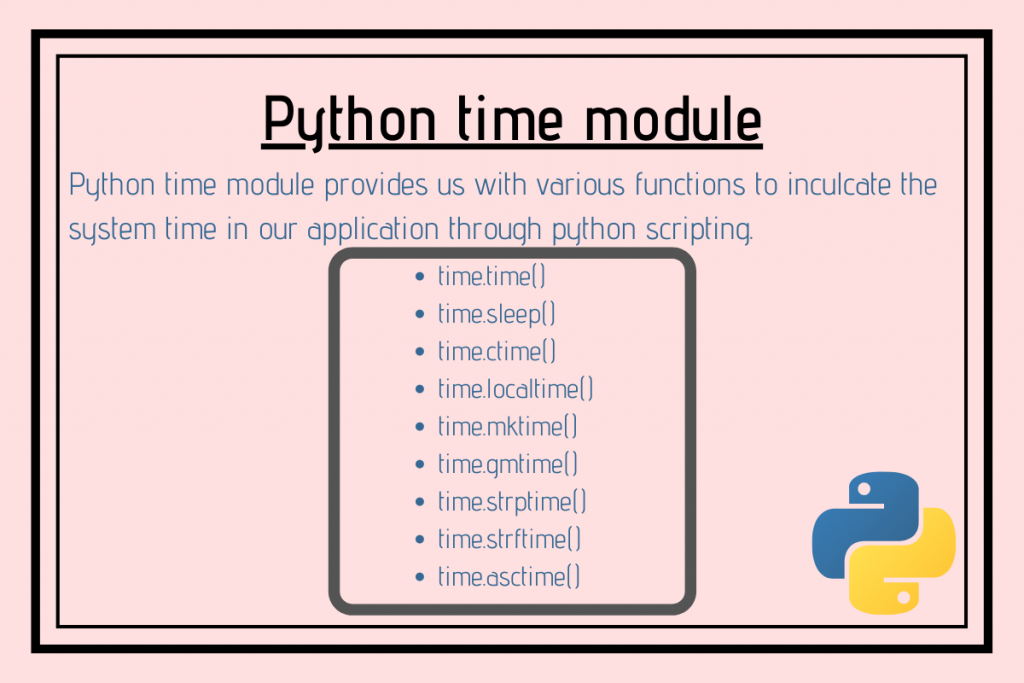# Python time modulePython time module provides us with various functions to inculcate the system time in our application through python scripting.

In order to get started with the time module, we need to import it in our python script using the below statement:

```import time
```

## Understanding the concept of ‘epoch’

While performing operations on the time stamp related data, it is necessary to have a starting point from wherein we can start performing operations on the same.

The epoch is basically the starting point of time from which the lapse of time is to be measured.

## Python time module functions

Python time module offers a good range of functions to deal with the time stamp.

Following are the most commonly used functions of the time module:

• time.time()
• time.sleep()
• time.ctime()
• time.localtime()
• time.mktime()
• time.gmtime()
• time.strptime()
• time.strftime()
• time.asctime()

### 1. time.time() method

Python time module has got `time.time()` method that gives the seconds of the current local time.

Syntax:

```time.time()
```

Example:

```import time
seconds = time.time()
print("Current time in seconds since epoch =", seconds)
```

Output:

```Current time in seconds since epoch = 1582961644.3032079
```

### 2. time.sleep() method

The time.sleep() method provides time-lapse or delay between the execution of the current processes or threads.

Syntax:

```time.sleep(value)
```

Example:

```import time

print("JournalDev!!!!.")
time.sleep(1.2)
time.sleep(3.2)
print("Engineering")
```

In the above snippet of code, when we try to execute the above code, one can easily observe the delay while the output statements are being displayed onto the console.

Output:

```JournalDev!!!!.
Engineering
```

### 3. time.localtime() method

The Python time module contains struct_time class which can be accessed with various functions of the time module. It helps us access the various fields of local time-stamp such as year, hour, seconds, etc.

The struct_time class consists of the following attributes:

• tm_year: Returns the year of the particular local time.
• tm_hour: Returns the hour of the particular local time.
• tm_min: Returns the minute value of the particular local time.
• tm_sec: Returns the seconds value of the particular local time.
• tm_mon: Returns the month of the local time.
• tm_mday: Returns the date of the month of the local time.
• tm_wday: Returns the value of the weekdays i.e. 0-Monday to 6-Sunday.
• tm_yday: Returns the number of the particular day ranging from 1-366

The `time.localtime()` function runs the time.time() function in back-end and returns the current time details in the format of the struct_time class in local time.

We can also pass the number of seconds since epoch as an argument to the function.

Syntax:

```time.localtime(seconds)
```

Example:

```import time

local_time = time.localtime()
print("Time:",local_time)
print("Current year:", local_time.tm_year)
print("Current hour:", local_time.tm_hour)
print("Current minute:", local_time.tm_min)
print("Current second:", local_time.tm_sec)
```

Output:

```Time: time.struct_time(tm_year=2020, tm_mon=2, tm_mday=29, tm_hour=14, tm_min=3, tm_sec=23, tm_wday=5, tm_yday=60, tm_isdst=0)
Current year: 2020
Current hour: 14
Current minute: 3
Current second: 23
```

### 4. time.ctime() method

The `time.ctime()` method takes the seconds value since epoch or the result of the time() function as an argument and returns a string value representing the current local time.

Syntax:

```ctime(seconds)
```

Example:

```from time import time, ctime

current_time = time()
res = ctime(tim)
print("Local_time:",res)
```

Output:

```Local_time: Sat Feb 29 14:08:26 2020
```

### 5. time.mktime() method

The `time.mktime()` method is the inverse of time.localtime() method.

It takes the struct_time (all the tuples of the struct_time class) as an argument and returns the time in seconds that has lapsed/passed since the epoch.

Syntax:

```time.mktime()
```

Example:

```import time

local_time = time.localtime()
sec = time.mktime(local_time)
print(sec)
```

In the above example, we have used the locatime() method to get the tuples of the struct_time class and have passed the same to the mktime() method.

Output:

```1582966721.0
```

### 6. time.gmtime() method

The `time.gmtime()` function runs the time.time() function in back-end and returns the current time details in the format of the struct_time class in UTC.

Syntax:

```time.gmtime()
```

Example:

```import time

local_time = time.gmtime()
print(local_time)
```

Output:

```time.struct_time(tm_year=2020, tm_mon=2, tm_mday=29, tm_hour=9, tm_min=2, tm_sec=49, tm_wday=5, tm_yday=60, tm_isdst=0)
```

### 7. time.strptime() method

The `time.strptime()` method accepts a string that represents the time and returns the time details in the struct_time format.

Syntax:

```time.strptime(string, %format code)
```

Format codes:

• %m – month
• %d – day
• %M – month
• %S – second
• %H – hour
• %Y – year

Example:

```import time

tym = "29 February, 2020"
sys = time.strptime(tym, "%d %B, %Y")
print(sys)
```

Output:

```time.struct_time(tm_year=2020, tm_mon=2, tm_mday=29, tm_hour=0, tm_min=0, tm_sec=0, tm_wday=5, tm_yday=60, tm_isdst=-1)
```

### 8. time.strftime() method

The `time.strftime()` method is the inverse of time.strptime() method. It takes tuples of struct_time class as an argument and returns a string representing the time according to the input format codes.

Syntax:

```time.strftime(%format code, struct_time)
```

Example:

```import time

tym = time.localtime()
opt = time.strftime("%d/%m/%Y, %H:%M:%S",tym)

print(opt)
```

Output:

```29/02/2020, 15:07:16
```

### 9. time.asctime() method

The `time.asctime()` method takes tuples of struct_time class as an argument and it returns a string representing the time input from the struct_time class tuples.

Example:

```import time

tym = time.localtime()

opt = time.asctime(tym)
print("TimeStamp:",opt)
```

Output:

```TimeStamp: Sat Feb 29 15:27:14 2020
```

## Conclusion

In this article, we have understood the Python time module and various functions offered by the same.

## References

Python time module